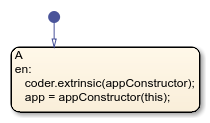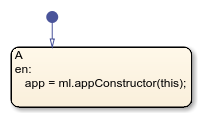# this

Access chart data during simulation

## Syntax

``this``

## Description

example

````this` provides external MATLAB® code, such as functions and apps, access to chart data during simulation. For charts in Simulink® models, external MATLAB code can access inputs, outputs, and local data.For standalone charts in MATLAB, external MATLAB code can access local data and call `step`, input event functions, and graphical and MATLAB functions in the chart. For more information, see Execute a Standalone Chart. NoteIn charts in Simulink models, the keyword `this` is supported only as an argument to external MATLAB code. Any other use of the keyword in the chart results in a compile-time error. ```

## Examples

expand all

Create a bidirectional connection between a Stateflow® chart and a MATLAB app created in App Designer. Call the app as an extrinsic function using `this` as an argument to the constructor. In the app, create a custom property to interface with the chart during simulation. In the chart, store the value returned by the function call to the app as a local data object.

In a chart that uses MATLAB as the action language, enter:

```coder.extrinsic(appConstructor); app = appConstructor(this);```In a chart that uses C as the action language, enter:

`app = ml.appConstructor(this);`For additional examples that illustrate this workflow, see Model a Power Window Controller and Simulate a Media Player.

Modify the value of the local data `x` while debugging a standalone Stateflow chart in MATLAB.

At the debugging prompt, enter:

`this.x = 7`

Note

When debugging a chart in a Simulink model, you can access all Stateflow data directly at the debugging prompt. For more information, see View and Modify Data in the MATLAB Command Window.

## Tips

• Do not use the keyword `this` to access chart data after simulation has stopped.

• Calling an external function named `this` from a chart disables the keyword `this` throughout the chart. To use the keyword, rename the extrinsic function.

(MATLAB Coder)

Introduced in R2020b

## SupportGet trial now